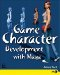# Hand Optimization

Kila's hands at this point do house a lot of detail, so reducing them by 151 polygons each should not significantly reduce their quality. We should be able to have all five fingers articulated, without having to resort to a mitten hand or one with frozen fingers.

So what's available for optimization? We can start by removing the fingernails. Here we have to remember that when working on the fingers, if we alter one we must do the same on the other four. They all have to match or they will look odd.

1.

On all the fingernails and the thumbnail, snap the inner vertices of the nails to the outer ones and weld them, as demonstrated in the before-and-after shots in Figure 5.54.

##### Figure 5.54. Start by removing the fingernails.Currently we have fewer divisions of faces on the wrist than we have across the back and palm of the hand. So we should next optimize the back of the hand. Figure 5.55 (left) shows the back of the hand currently.

##### Figure 5.55. Optimize the back of the hand so it matches the divisions on the wrist.2.

Following the center of the X created for the knuckles, trace back to the two vertices behind it and weld them to the ones on their left (Figure 5.55, right).

3.

Next, flip the hand over and do the same for the palm, reducing it to match the divisions on the wrist. Figure 5.56 shows the palm before and after the optimization.

##### Figure 5.56. Reduce the palm as you did the back of the hand.4.

You might be left with the two edges I have highlighted in Figure 5.57 (left). If so, you can simply collapse these because they don't add anything to the model.

##### Figure 5.57. Collapse these two edges if they exist on your model.The main hand area is now done, but we can reduce it even further by optimizing the fingers.

1.

Flatten the top of each finger as shown in Figure 5.58. Do this by snapping each of the vertices that run down the center to the ones directly to their right. Remember to weld them afterward, or the polygons will still exist.

##### Figure 5.58. Flatten the top of each finger.2.

Right at the tip of each finger there is a single vertex that we no longer need. It doesn't add much to the shape, so snap it to the vertex at the tip of the nail, and weld (Figure 5.59).

##### Figure 5.59. Remove the vertex in the center of each fingertip.3.

Still looking at the fingertip, there are two edges at either side of the nail; you can see these in Figure 5.60, left. Collapsing these on each finger will recoup another 20 polygons.

##### Figure 5.60. Collapse the two edges on each side of the fingernail.That completes the first stage of reducing the hand; you can see the results in Figure 5.61. We could leave it as-is, but there's more we can do. I don't think we will see a major difference in quality if we move forward one more step, which will reduce the polygon count by another 160.

##### Figure 5.61. The hand model so far4.

As we did on the top of the hand, we can optimize the sides of the fingers and thumb. Select the vertices that run down both sides of each digit, and snap and then weld them to the vertices below. Figure 5.62 shows you how this looks on just the index finger.

##### Figure 5.62. Flatten the sides of each finger and thumb.NOTE

This operation not only removes the side-finger divisions but also reduces the number of mesh divisions on the underside of each knuckle from 3 to 1.

We will leave the bottom curve of the fingers intact. This will provide a little more shape than they would have if we reduced them to cubes.

Once all the digits have been done, the hand is finished (Figure 5.63).

##### Figure 5.63. Final optimized hand modelGame Character Development with Maya
ISBN: 073571438X
EAN: 2147483647
Year: 2004
Pages: 169
Authors: Antony Ward

Similar book on Amazon